Study Guide

# Similar Triangles - Similarity in Other Polygons

## Similarity in Other Polygons

Triangles, triangles, triangles! Why do they get all the attention?

It's so hard being the younger sibling of the strongest and most stable shape there is. How about some love for the rest of the shapes? Can't we talk about any of them being similar, too?

Well, sure. It turns out all circles are similar. So are all squares. And if a pentagon, hexagon, octagon, or any other n-gon is regular, then it's similar to all of its regular like-sided n-gons as well. Just like equilateral triangles.

### Sample Problem

Determine if these two polygons are similar.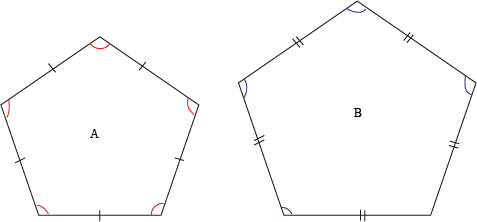We are given that in pentagon A, the five sides and five angles are all congruent to each other, which makes it a regular pentagon. Same for pentagon B.

Since regular polygons with the same number of sides are always similar, we can determine that pentagon A is similar to pentagon B.Two irregular shapes could be similar, but there's no quick rule about them, so we'll have to check that all of their corresponding side lengths are proportional and all of their corresponding angles are congruent.

### Sample Problem

Determine whether these quadrilaterals are similar.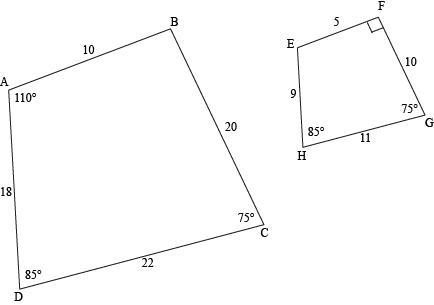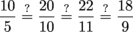Yep, all of those ratios are equivalent, so we're good with proportional side lengths.

The measures of ∠B and ∠E are missing, so we'll need to calculate them. Let's remember that the sum of the interior angles of all quadrilaterals is 360°.

m∠B = 360 – 110 – 75 – 85 = 90

Since ∠F is labeled as a right angle, this looks promising. But we'll double-check ∠E to be sure.

m∠E = 360 – 90 – 75 – 85 = 110

This matches the measure of corresponding angle ∠A. Four congruent angles. Check and check. They're similar.

Why do we need to check the sides and angles in our shapes? Isn't it good enough to check just one of the requirements?

Sadly, nope. Let's consider these examples:

These quadrilaterals have proportional side lengths, but their angles are definitely not congruent.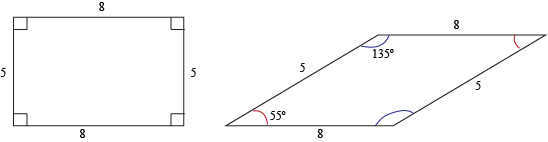Meanwhile, these pentagons have congruent angles, but their side lengths are definitely not proportional.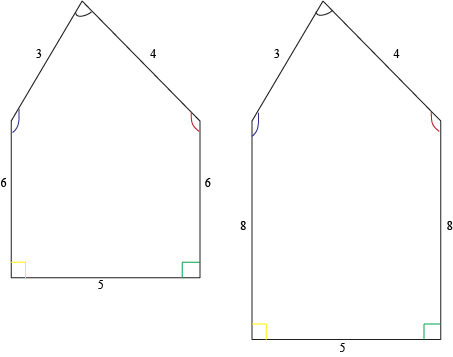Of course, if the first thing you test (either side lengths or angles) doesn't meet its requirement (proportional or congruent, as the case may be), you don't need to bother checking the other, since both are required for the shapes to be similar. But if the first one comes back positive, you ought to go ahead and test the other.

Think of it like this: when it comes to similar polygons, there's no such thing as a false negative, but there are false positives. And if you make decisions based on false positives, you may find yourself sorely disappointed.

### Sample Problem

Determine if these two rectangles are similar.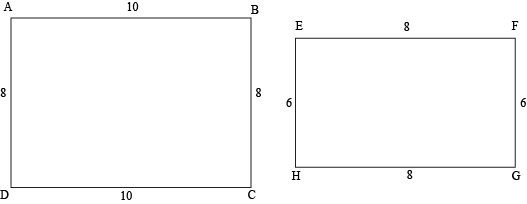We're given the fact that the shapes are rectangles, which, by definition, have four right angles. So we know that they meet the congruent angles requirement.

We can write ratios to compare the side lengths of corresponding sides, then check to see if they're proportional.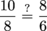Nope, those ratios aren't equivalent, so the sides aren't proportional. These rectangles aren't similar.

### Sample Problem

Determine if these polygons are similar.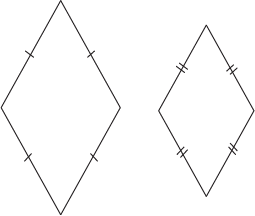Let's consider the side lengths first. In each quadrilateral, the four sides are congruent. That's going to make the ratios of side lengths proportional. But what about the angles? Frankly, the problem doesn't give us enough information to make the call. The angles sure look congruent, but appearances can be deceiving, and since this drawing isn't labeled "drawn to scale," it wouldn't even matter if we grabbed our protractors and measured them.

Just for fun, similar polygons also have proportional corresponding diagonals. (As if congruent angles and proportional sides weren't enough!)

### Sample Problem

ABCD ~ EFGH. Find the value of x.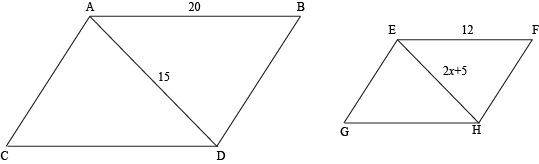Since diagonals are proportional, just like the side lengths, we can set up and solve a proportion to find the length of diagonal EH, and then the value of x.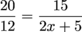20(2x + 5) = 12 × 15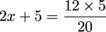2x + 5 = 9
2x = 4
x = 2